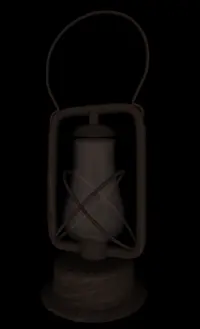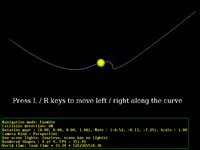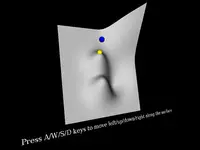# NURBS component

This component defines nodes for rendering and animating along smooth NURBS curves and surfaces.Contents:

## 1. Demos

For demos and tests of these features, see the `nurbs` subdirectory inside our VRML/X3D demo models.

## 2. X3D support

Full support for `NurbsPatchSurface`(Pascal API: `TNurbsPatchSurfaceNode`),
`NurbsCurve`(Pascal API: `TNurbsCurveNode`),
`NurbsPositionInterpolator`(Pascal API: `TNurbsPositionInterpolatorNode`),
`NurbsSurfaceInterpolator`(Pascal API: `TNurbsSurfaceInterpolatorNode`),
`NurbsOrientationInterpolator`(Pascal API: `TNurbsOrientationInterpolatorNode`).

Any >= 2 value of order is allowed (X3D spec requires only 2,3,4 support).

`NurbsTrimmedSurface`(Pascal API: `TNurbsTrimmedSurfaceNode`) has only partial support: it is rendered just like `NurbsPatchSurface`, ignoring `trimmingContour`.

## 3. Differences from NURBS nodes defined in VRML 97 Amendment 1

Since 2022-09-01, we no longer support NURBS specifics defined in old VRML 97 Amendment 1 specification. Instead you should use standard X3D NURBS nodes (even inside older VRML 2.0 / 97).

Note that VRML 97 Amendment 1 NURBS nodes are similar, but not 100% the same as their X3D counterparts. You should adjust the NURBS usage in your VRML files to X3D standard (or, even better, just upgrade your whole models to latest X3D standard). Differences:

• Only X3D surfaces have `xClosed` fields. We treat TRUE value there as "possibly closed", that is — if field indicates closed, we still check if limiting points match (X3D spec suggests we should do this, as far as I understand). This means X3D models may be loaded faster — if xClosed = FALSE, we do not even check if limiting points match.

• Only VRML 97 surfaces have `ccw` field.

• VRML 97 versions specify `coord` as direct `MFVec3f` field, while X3D versions specify `coord` as `SFNode` (containing `Coordinate` or similar node).

• VRML 97 `NurbsPositionInterpolator` has different field names (keyValue, keyWeight, following interpolator conventions) than X3D `NurbsPositionInterpolator` (controlPoint, weight, following nurbs conventions).

• VRML 97 `NurbsPositionInterpolator` has different default value for order (4) than X3D version (3). Beware of this when converting from VRML 97 to X3D.

• In VRML 97, `knot` and `weight` data is `MFFloat`, single-precision. In X3D, it's `MFDouble`.

## 4. Control points are in homogeneous coordinates

Note that in VRML and X3D, NURBS control points are expressed in homogeneous coordinates. That is, the control point is actually a 4D vector `(x, y, z, weight)`, which means that it's actual 3D position is `(x/weight, y/weight, z/weight)`.

This may be a little confusing, if you're used to normal NURBS equation definition like from here or at Wikipedia. Instead of usual equation:

`P(u) = (sum of basis * control point * weight) / (sum of basis * weight)`

VRML/X3D uses a simpler equation:

`P(u) = (sum of basis * control point) / (sum of basis * weight)`

That is, "X3D control point" (as specified in VRML/X3D file) is assumed to be already multiplied by weight.

If you want to intuitively pull the curve toward the control point, you should

1. Calculate "normal control point" (3D, not in homogeneous coordinates) as "X3D control point / weight".
2. Increase the weight (to pull the curve toward "normal control point"), or decrease (to push the curve away from it).
3. Calculate new "X3D control point" as "normal control point * new weight".

In other words, if you just want to increase the weight 2 times, then the corresponding control point should also be multiplied * 2, to make things behave intuitive.

In particular, when writing an exporter from normal 3D modeling programs, like Blender, note that you have to multiply Blender control points * Blender weights to get correct X3D control points. When you use White Dune, a NURBS 3D modeler especially suited for working with VRML/X3D, this non-intuitive behavior is somewhat hidden (the curve "handles" you see in White Dune are actually "X3D control points / divided by weight").

Our behavior is compatible with other X3D browsers/editors (at least White Dune, Octaga, InstantPlayer, BitManagement).